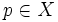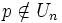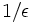# Metrizable implies perfectly normal

This article gives the statement and possibly, proof, of an implication relation between two topological space properties. That is, it states that every topological space satisfying the first topological space property (i.e., metrizable space) must also satisfy the second topological space property (i.e., perfectly normal space)
View all topological space property implications | View all topological space property non-implications
Get more facts about metrizable space|Get more facts about perfectly normal space

## Statement

Any metrizable space, i.e., any space realized as the topological space for a metric space, is a perfectly normal space -- it is a normal space and every closed subset of it is a G-delta subset (it is a countable intersection of open subsets).

## Facts used

1. Metrizable implies normal

## Proof

Given: A metric space$(X,d)$. with the topology arising from the metric.

To prove:$X$ is a perfectly normal space:$X$ is a normal space and for every closed subset$A$ of$X$, there is a countable collection of open subsets$U_n$ of$X$ such that$A$ equals the intersection of the$U_n$s.

Proof: By fact (1),$X$ is a normal space, so we show the second part of the definition. For the closed subset$A$, define$U_n$ as the set of all points$p \in X$ such that there exists a point$a \in A$ such that$d(p,a) < (1/n)$. Then:

1. Each$U_n$ is open:$U_n$ is the union of the open balls of radius$1/n$ about all the points of$A$. Hence, it is a union of open subsets, hence open.
2. The intersection of the$U_n$s contains$A$.
3. If$p$ is not in$A$, there is some$U_n$ such that$p \notin U_n$: Since$A$ is closed, there exists$\epsilon$ such that the ball of radius$\epsilon$ about$p$ does not intersect$A$. In other words, there is no point of$A$ whose distance from$p$ is less than$\epsilon$. Let$n$ be a positive integer greater than$1/\epsilon$. Then,$U_n$ does not contain$p$.

Together, (1), (2) and (3) complete the proof.